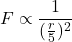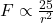## The separation between two magnets is reduced by a factor of 5. How does the force between them change? Question 18 options:

Question

The separation between two magnets is reduced by a factor of 5. How does the force between them change?

Question 18 options:

increases by a factor of 5

increases by a factor of 25

decreases by a factor of 5

decreases by a factor of 25

doesn’t change

in progress 0
6 months 2021-07-17T12:21:55+00:00 1 Answers 4 views 0

1. Answer:Increase by a factor of  25

Explanation:

We know that magnetic force is proportional towhere r is the distance between them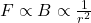Thus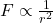Where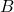=magnetic field

If distance is reduced by a factor of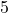then force must increase by a factor of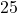because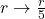so